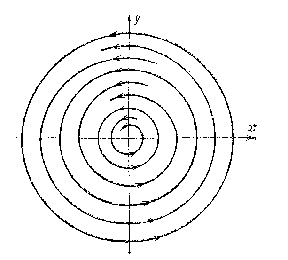# Centro-focus

A kind of pattern of the trajectories of an autonomous system of ordinary second-order differential equations (*) in a neighbourhood of an isolated singular point $x _ {0}$, where

$$\tag{* } \dot{x} = f ( x),\ \ x \in \mathbf R ^ {2} ,\ \ f: G \rightarrow \mathbf R ^ {2} ,$$

$f \in C ( G)$ and $G$ is a domain of uniqueness. This type is characterized as follows: In every neighbourhood $U$ of $x _ {0}$ there exist closed trajectories of the system going around $x _ {0}$ and also complete trajectories that are not closed; the latter fill out annulus-shaped domains contracting to $x _ {0}$ and bounded by the closed trajectories; the trajectories in the interiors of an arbitrary annulus are spirals for which one end asymptotically approximates the outer boundary, while the other end asymptotically approximates the inner boundary of the annulus. The point $x _ {0}$ itself is also called a centro-focus.Figure: c021320a

In the figure, $( 0, 0)$ is a centro-focus; the arrows indicate the direction of motion along the trajectories of the system with increasing $t$( they may also go in the opposite direction).

A centro-focus is Lyapunov (but not asymptotically) stable. Its Poincaré index is 1.

How to Cite This Entry:
Centro-focus. Encyclopedia of Mathematics. URL: http://encyclopediaofmath.org/index.php?title=Centro-focus&oldid=53347
This article was adapted from an original article by A.F. Andreev (originator), which appeared in Encyclopedia of Mathematics - ISBN 1402006098. See original article